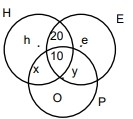### CAT 2019 – Slot 1 – Quantitative Ability – If log(base12)⁡ 81=p

Q. 1: If log(base12)⁡ 81=p then 3 { (4-p)/(4+p)} is equal to
1. log (base2) 8
2. log (base4) 16
3. log (base6) 8
4. log (base6) 16

Given , log(base12)⁡ 81 = p

4 log (base12) 3 = p

So 3 (4-p)/(4+p) = 3 (1- log(base12)⁡ 3 )/(1+ log(base12)⁡ 3)=3 × log(base12)⁡(12/3)/log(base12)⁡(12×3) = 3×log(base36)⁡ 4 = log(base6) ⁡8

##### Q. 2: Train T leaves station X for station Y at 3 pm. Train S, traveling at three quarters of the speed of T, leaves Y for X at 4 pm. The two trains pass each other at a station Z, where the distance between X and Z is three-fifths of that between X and Y. How many hours does train T take for its journey from X to Y?

Train T starts at 3 PM and train S starts at 4 PM. Let the speed of train T be t.

=> Speed of train S = 0.75t.

When the trains meet, train t would have traveled for one more hour than train S.

Let us assume that the 2 trains meet x hours after 3 PM. Trains S would have traveled for x-1 hours.

Distance traveled by train T = xt

Distance traveled by train S = (x-1)*0.75t = 0.75xt-0.75t

We know that train T has traveled three fifths of the distance. Therefore, train S should have traveled two-fifths the distance between the 2 cities.

=> (xt)/(0.75xt-0.75t) = 3/2

2xt = 2.25xt-2.25t 0.25x = 2.25

x = 9 hours.

Train T takes 9 hours to cover three-fifths the distance. Therefore, to cover the entire distance, train T will take 9*(5/3) = 15 hours.

Therefore, 15 is the correct answer.

Q. 3: Each of 74 students in a class studies at least one of the three subjects H, E and P. Ten students study all three subjects, while twenty study H and E, but not P. Every student who studies P also studies H or E or both. If the number of students studying H equals that studying E, then the number of students studying H is

Let the number of students who studying only H be h, only E be e, only H and P but not E be x, only E and P but not H be yGiven only P = 0 All three = 10; Studying only H and E but not P = 20 Given number of students studying H = Number of students studying E

= h + x + 20 + 10

= e + y + 20 + 10

h + x = e + y total number of students = 74 Therefore, h + x + 20 + 10 + e + y = 74

h + x + e + y = 44 h + x + h + x = 44 h + x = 22

Therefore, the number of students studying H = h + x + 20 + 10 = 22 + 20 + 10 = 52.

Q. 4: A wholesaler bought walnuts and peanuts, the price of walnut per kg being thrice that of peanut per kg. He then sold 8 kg of peanuts at a profit of 10% and 16 kg of walnuts at a profit of 20% to a shopkeeper. However, the shopkeeper lost 5 kg of walnuts and 3 kg of peanuts in transit. He then mixed the remaining nuts and sold the mixture at Rs. 166 per kg, thus making an overall profit of 25%. At what price, in Rs. per kg, did the wholesaler buy the walnuts?
1. 98
2. 96
3. 84
4. 86

let the CP of walnuts for wholesaler was 3x and that of peanuts was x so

SP of 8 kg of peanuts and 16 kg of walnuts = 8*1.1x + 16*1.2*3x = 66.4x

The shopkeeper gets 25% profit so SP = 1.25*66.4x =83x

As per question 83x = 166*(8+16 – 5-3) = 166*16

So x = 32

So CP of walnuts = 3x = 96

Q. 5: A CAT aspirant appears for a certain number of tests. His average score increases by 1 if the first 10 tests are not considered, and decreases by 1 if the last 10 tests are not considered. If his average scores for the first 10 and the last 10 tests are 20 and 30, respectively, then the total number of tests taken by him is

let total number test taken by him = n and average score = x

So average score of last (n-10) test = x+1

Sum of scores of last n-10 test = (n-10)*(x+1) = nx – ( 10x – n + 10)

So total score of first 10 test = 10x – n + 10

20*10 = 10x – n + 10

10x – n = 190  —————1)

average score of first (n-10) test = x-1

Sum of scores of first n-10 test = (n-10)*(x-1) = nx – ( n +10x – 10)

So total score of first 10 test = ( n +10x – 10)

30*10 = ( n +10x – 10)

n + 10x = 310 —————2)

by solving eq 1 ) & eq 2)

n = 60, x = 25

so total number of test taken by him = 60

Inspiring Education… Assuring Success!!

Ghatkopar | Borivali | Andheri | Online